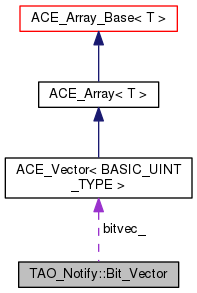TAO_Notify::Bit_Vector Class Reference

Simple bit vector. More...

`#include <Bit_Vector.h>`

Collaboration diagram for TAO_Notify::Bit_Vector:[legend]

## Public Member Functions

Bit_Vector ()
The constructor. More...

~Bit_Vector ()
The destructor. More...

bool is_set (const size_t location) const
Determine if a bit at location is set. More...

void set_bit (const size_t location, bool set)
Set or unset a bit at location, growing the vector as needed. More...

size_t find_first_bit (bool set) const
Find the first bit that is either set or unset in an O(1). More...

## Private Types

enum  { BITS_PER_WORD = 32, BPW_LOG_2 = 5 }

typedef ACE_UINT32 BASIC_UINT_TYPE

typedef ACE_Vector< BASIC_UINT_TYPEVECTOR_TYPE

## Private Member Functions

void evaluate_firsts (const size_t location, bool set)
Update our first set and unset bits. More...

size_t find_first_bit_of (const size_t location, bool set)

## Private Attributes

VECTOR_TYPE bitvec_

size_t size_

size_t first_set_bit_

size_t first_cleared_bit_

## Detailed Description

Simple bit vector.

Written to support block allocation from persistent storage. Should be promoted to the ACE level to make it generally usable.

## Member Typedef Documentation

 typedef ACE_UINT32 TAO_Notify::Bit_Vector::BASIC_UINT_TYPE
private
 private

## Member Enumeration Documentation

 anonymous enum
private
Enumerator
BITS_PER_WORD
BPW_LOG_2

## Constructor & Destructor Documentation

 TAO_Notify::Bit_Vector::Bit_Vector ( )

The constructor.

 TAO_Notify::Bit_Vector::~Bit_Vector ( )

The destructor.

## Member Function Documentation

 void TAO_Notify::Bit_Vector::evaluate_firsts ( const size_t location, bool set )
private

Update our first set and unset bits.

 size_t TAO_Notify::Bit_Vector::find_first_bit ( bool set ) const

Find the first bit that is either set or unset in an O(1).

 size_t TAO_Notify::Bit_Vector::find_first_bit_of ( const size_t location, bool set )
private

Iterate from location to the end, finding the first bit that matches the requested set or unset value.

 bool TAO_Notify::Bit_Vector::is_set ( const size_t location ) const

Determine if a bit at location is set.

 void TAO_Notify::Bit_Vector::set_bit ( const size_t location, bool set )

Set or unset a bit at location, growing the vector as needed.

## Member Data Documentation

 VECTOR_TYPE TAO_Notify::Bit_Vector::bitvec_
private
 size_t TAO_Notify::Bit_Vector::first_cleared_bit_
private
 size_t TAO_Notify::Bit_Vector::first_set_bit_
private
 size_t TAO_Notify::Bit_Vector::size_
private

The documentation for this class was generated from the following files: